## 堆和堆排序 转

o
osc_a22drz29

### 一、什么是优先队列？

 入队 出队 普通数组 O(1) O(n) 顺序数组 O(N) O(1) 堆 O(logN) O(logN)

### 二、堆的基本实现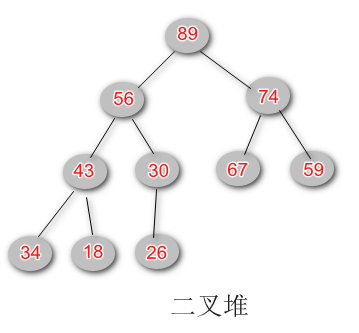• 任意节点小于其父节点
• 除了最后一层叶子节点外，其他层的元素个数必须是最大值 ，叶子节点虽然可以不是最大值，但必须靠左排列(最大堆)
• 堆是一棵完全二叉树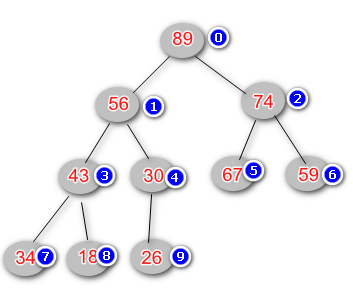• 父节点下标：parent (i) = ( i - 1) / 2
• 左子节点： left child (i)  = (i + 1) * 2
• 右子节点：right child (i) = (i + 1) * 2 + 1

`````` 1 public class MaxHeap {
2
3     // 存储元素
4     private int[] data;
5     // 记录堆中节点个数
6     private int size;
7     // 堆的容量
8     private int capacity;
9
10     public MaxHeap(int capacity) {
11
12         this.capacity = capacity;
13         data = new int[capacity]; // 堆的第一个元素索引为 0;
14         this.size = 0;
15     }
16
17     public int size() {
18         return size;
19     }
20
21     public boolean isEmpty() {
22         return size == 0;
23     }
24
25 }``````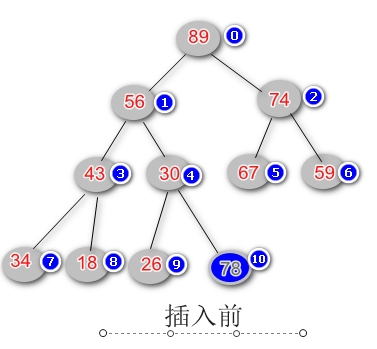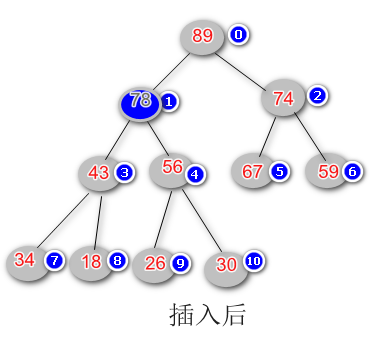``````/**
* 往最大堆中加入一个元素
* @param e
*/
public void insert(int e) {

if (size - 1 < capacity) {
data[size] = e;
shiftUp(size);
size++;
}
}

/**
* 根据堆的定义，交换父子节点的位置，直到满足最大堆的原则
* @param k
*/
private void shiftUp(int k) {

while (k > 0 && data[k] > data[(k - 1) / 2]) {
SortedHandler.swap(data, k, (k - 1) / 2);
k = (k - 1) / 2;
}
}``````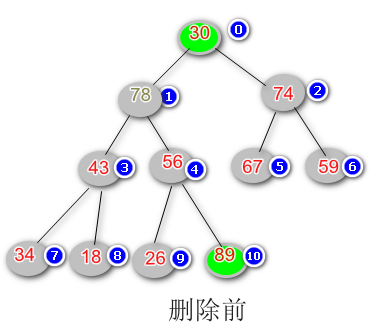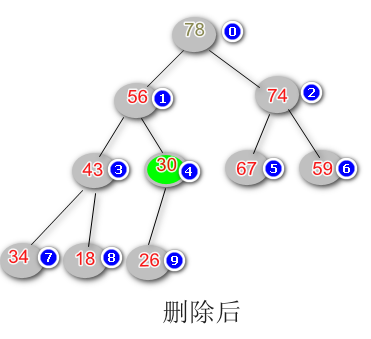根据优先队列的定义，元素的出顺序按照优先级，而在最大堆中，根节点的优先级就是最大的，因此我们删除的时候，总是从根节点开始。

具体的思路是，首先交换根节点和最后一个节点的位置，然后删除掉交换后的根节点，也就是最大值，然后根据堆的定义交换节点位置维护最大堆的原则，最后返回删除了的最大值即可。代码实现如下：

``````/**
* 交换根节点和最后一个节点的位置，再将移除的根节点的值返回，并维护最大堆的原则
* @return 原堆中的最大值
*/
public int extractMax() {

if (size > 0) {
int res = data;

// 交换第一个和最后一个的位置
SortedHandler.swap(data, 0, size - 1);
size--;
shiftDown(0);
return res;
}

return -1;
}

/**
* 交换节点的位置  维护最大堆的定义
* @param k 开始的节点位置
*/
private void shiftDown(int k) {

while (2 * k + 1 < size) {
int j = 2 * k + 1; // 左子点的下标
if (j + 1 < size && data[j + 1] > data[j]) {
j += 1;
}
if (data[k] < data[j]) {
SortedHandler.swap(data, k, j);
k = j;
} else {
break;
}
}

}``````

### 三、基本的堆排序

通过上面的努力，我们实现了一个基本操作的最大堆。如果前面的明白了的话，那么实现一个堆排序就是小问题了，因为我们的最大堆的输出顺序就是由大到小的，那么排序的问题不过是将数组的顺序反过来 .

``````public static void heapSorted1(int arr[]) {

int n = arr.length;
MaxHeap heap = new MaxHeap(n);

for (int i = 0; i < n; i++) {
heap.insert(arr[i]);
}
for (int i = n - 1; i >= 0; i--) {
arr[i] = heap.extractMax();
}
}``````

最大堆的另外一种构造方法 —— Heapify

在前面构造最大堆的实现中，我们都是首先构造一个初始化容量的数组，然后依次加入数组的每个元素。现在我们考虑一个情况，因为最大堆的存储本身就是数组实现的，那么当我们对数组需要排序的时候，是否可以直接将这个数组构造成为最大堆呢，而无需逐个的复制元素并shiftUp？答案是肯定的。

具体的思路是：将待排序的数组本身看成是一棵二叉树，在这课二叉树中，所有不同的非叶子节点就是不用的最大堆。那么我们就从这棵二叉树的第一个非叶子节点开始执行shiftDown操作，直到整棵二叉树满足最大堆的原则。那么问题又来了？第一个非叶子是多少呢，这里又有一个规律：完全二叉树的排列中，第一个非叶子节点 i 等于数组的长度 (n - 1) / 2.代码实现如下：

``````// heapify 的过程
public MaxHeap(int arr[]) {

int n = arr.length;
data = new int[n];
capacity = n;
for (int i = 0; i < n; i++) {
data[i] = arr[i];
}
size = n;
// 第一个非叶子节点的下标 (n-1) / 2
for (int i = (n - 1) / 2; i >= 0; i--) {
shiftDown(i);
}

}

public static void heapSorted2(int arr[]) {

int n = arr.length;
MaxHeap heap = new MaxHeap(arr);

for (int i = n - 1; i >= 0; i--) {
arr[i] = heap.extractMax();
}

}``````

### 四、堆排序的优化——原地堆排序

``````public static void heapSorted3(int arr[]) {

int n = arr.length;

// heapify ,将数组转换为堆
for (int i = (n - 1) / 2; i >= 0; i--) {
__shiftDown(arr, n, i);
}

//
for (int i = n - 1; i > 0; i--) {
int tmp = arr[i];
arr[i] = arr;
arr = tmp;

__shiftDown(arr, i, 0);
}

}

/**
*  原地堆排序的shiftDown操作
* @param arr
* @param n
* @param i
*/
private static void __shiftDown(int[] arr, int n, int i) {

while (2 * i + 1 < n) {
int j = 2 * i + 1;
if (j + 1 < n && arr[j] < arr[j + 1]) {
j += 1;
}
if (arr[j] > arr[i]) {
int tmp = arr[j];
arr[j] = arr[i];
arr[i] = tmp;

i = j;
} else
break;
}

}``````
o

### osc_a22drz29#### 暂无文章

48分钟前
10
0
unity列表控件Horizontal/Vertical/Grid Layout Group用法介绍

1. Grid Layout Group 为Panel控件添加Grid Layout Group，子控件为四个按钮，分别为Grid，Calendar，Gear，User： 默认属性为 为方便演示，按钮的底色为控件自带image，按钮上面的图标为其子...

19
0
Distinct（）与lambda？ - Distinct() with lambda?

16
0

17
0
OSChina 周一乱弹 —— 毛巾又怎么样？！我在乎的是大姐姐温柔的怀抱！

Osc乱弹歌单（2020）请戳（这里） 【今日歌曲】 @薛定谔的兄弟 ：分享洛神有语创建的歌单「我喜欢的音乐」: 《雨 因你而下，于你而止》- Seto 手机党少年们想听歌，请使劲儿戳（这里） @Dan...

48
2